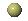# Solving Danzig, Fulkerson and Johnson's original 48 city TSP instance by Linear Programming using CPLEX

## Historical Perspective

The Traveling Salesman Problem or TSP, can be stated as follows: Given n "cities" along with the cost of travel between them, find the cheapest way of visiting all cities and returning to your starting point. This problem is well know to be NP-Complete, and is extremely difficult to solve for even small values of n; a brute force algorithm would have go through a search space of order O(n!), which makes this approaches totally infeasible all but for the smallest values of n. Danzig, Fulkerson and Johnson were the first to solve a non-trivially sized instance of the TSP. This was accomplished in 1954, when they solved a 48 city instance using Linear Programming techniques. A linear program can be described succinctly as:
Maximize (or Minimize) cx subject to the system of inequalities,
Ax <= b
This type of problem can usually be solved efficiently using the Simplex method. Exactly how Danzig, Fulkerson and Johnson used linear programming to solve their 48 city instance is described in a paper I wrote in the context of the undergraduate course 308-566 Computer Methods in Operations Research taught at McGill University. Besides going their solution, the paper puts in perspective the evolution and the importance of the techniques and paradigms developed while solving the 48 city TSP instance.

## Solving the 48 city TSP Instance using CPLEX

In order to experience for myself the glorious moments and the satisfaction of solving a large scale TSP problem using linear programming, I set out to solve Danzig, Fulkerson and Johnson's quintessential 48 city TSP instance using the exact same constraints as put forward in their paper. I used the software package CPLEX as my LP solver. To generate the actual linear program, I built a small C program that emits the LP in CPLEX syntax, generating all constraints and the objective function. The emitted LP instance is parametrized in terms of the travel costs variables; these variables are defined the file distances.h. Using the C preprocessor, the symbolic travel costs in the generated LP are replaced by their actual values as defined in distances.h. This yields the final version of the linear program which can then be feed to the CPLEX engine.
 The C program: lp_generator.c The travel cost file: distances.h A Makefile to automate the generation of the LP: Makefile The generated CPLEX LP instance: out.lp
To build the LP type:
make lp
To build an Integer Program version of the problem, type:
make ilp
Edit the Makefile if need be.

Here is the solution to the generated LP as found by CPLEX:

```
CPLEX> opt
Tried aggregator 1 time.
LP Presolve eliminated 16 rows and 0 columns.
Reduced LP has 51 rows, 861 columns, and 3751 nonzeros.
Presolve time =    0.01 sec.
Iteration log . . .
Iteration:     1    Infeasibility =            14.999999
Iteration:    26    Objective     =          1258.000000
Iteration:    94    Objective     =           738.500000

Primal - Optimal:  Objective =    6.9860000000e+02
Solution time =    0.04 sec.  Iterations = 118 (25)

CPLEX> di so va -
Variable Name           Solution Value
x_2_1                         1.000000
x_42_1                        1.000000
x_3_2                         1.000000
x_4_3                         1.000000
x_5_4                         1.000000
x_6_5                         1.000000
x_7_6                         1.000000
x_8_7                         1.000000
x_9_8                         1.000000
x_10_9                        0.800000
x_25_9                        0.200000
x_12_10                       0.400000
x_25_10                       0.800000
x_12_11                       1.000000
x_23_11                       0.400000
x_24_11                       0.600000
x_13_12                       0.600000
x_14_13                       1.000000
x_17_13                       0.400000
x_15_14                       1.000000
x_16_15                       1.000000
x_17_16                       0.600000
x_18_16                       0.400000
x_18_17                       0.600000
x_23_17                       0.400000
x_19_18                       1.000000
x_20_19                       1.000000
x_21_20                       1.000000
x_22_21                       0.600000
x_28_21                       0.400000
x_23_22                       1.000000
x_28_22                       0.400000
x_24_23                       0.200000
x_25_24                       0.200000
x_27_24                       1.000000
x_26_25                       0.800000
x_27_26                       1.000000
x_28_26                       0.200000
x_29_28                       1.000000
x_30_29                       1.000000
x_31_30                       1.000000
x_32_31                       1.000000
x_33_32                       1.000000
x_34_33                       1.000000
x_35_34                       1.000000
x_36_35                       1.000000
x_37_36                       1.000000
x_38_37                       1.000000
x_39_38                       1.000000
x_40_39                       1.000000
x_41_40                       1.000000
x_42_41                       1.000000
All other variables in the range 1-861 are zero.

```
The above solution is of course not a valid TSP tour, as some of the variables take on fractional values. Note that because all our TSP edge cost take on integral values, the cost of the tour must integral and hence the previous solution tells us the cost of the optimal tour is >= 699 since the solution obtained was 698,6. Now if we consider the tour:

```x_2_1   = 1
x_3_2   = 1
x_4_3   = 1
...
x_42_41 = 1
x_42_1  = 1
```

We find that it's cost is 699. We can therefore conclude that it is optimal by the preceding comments.

This is what we get if we solve the Integer generated version of the linear program. In this case here is the CPLEX solution.Cuts for Special Structure -Brief introduction to Comb inequalities in the context of the TSP.David Neto's TSP reading list -Pointers to latest TSP solving literature.Record traveling salesman solution -General audience article commenting on the solving of a record size 13,509 city TSP instance by Bixby, Applegate, Cook and Chvatal.The traveling salesman problem -V. Chvatal's TSP homepage, along with links to the C code used to solve the USA13509 and a related survey paper.DIMACS -Center for Discrete Mathematics and Theoretical Computer Science.

## PapersPapers on the TSP -List of various papers on the TSPProvably good solutions for the TSP -TSP solving techniques and experimental results.Finding Cuts in the TSP -Technical report on TSP solving techniques written by Applegate, Bixby, Chvatal and Cook.Solving the Traveling Salesman Problem with a Distributed Branch-and-Bound Algorithm
Patrice POMINVILLE